• 好久没有写文章了，抱歉了，以后每天...两个向量“相乘”：等于两个向量的模(长度)乘于夹角的余弦在二维空间中，向量的点乘：使用余弦定理证明：向量点乘的直观理解：向量的点乘，两个向量必须是同方向的，所以做...

好久没有写文章了，抱歉了，以后每天都会更新一篇的....

向量的点乘，也就是两个向量相乘：

我们是不这么定义的，不是两个向量对应的坐标元素相乘：两个向量“相乘”，结果是⼀个数！，两个向量"相乘"，更严格的说法：两个向量的点乘，两个向量的内积。两个向量“相乘”：等于两个向量的模(长度)乘于夹角的余弦在二维空间中，向量的点乘：使用余弦定理证明：向量点乘的直观理解：

向量的点乘，两个向量必须是同方向的，所以做投影以后的长度再相乘同样，可以用坐标来理解：

v向量分解为x轴的x2向量，y轴的y2向量，u向量分解为x轴的x1向量，和y轴的y1向量，然后分别相乘，有4种情况，垂直的向量相乘为0，所以是x1.x2+y1.y2使用Python实现向量的点乘：具体代码：

定义一个内部使用的文件_globals，用来存储全局使用的变量 EPSILON，用来判断精度用的EPSILON = 1e-8

Vector的代码：

import math

from ._globals import EPSILON

class Vector:

def __init__(self, lst):

self._values = list(lst)

@classmethod

def zero(cls, dim):

"""返回一个dim维的零向量"""

return cls( * dim)

"""向量加法，返回结果向量"""

assert len(self) == len(another), \

"Error in adding. Length of vectors must be same."

return Vector([a + b for a, b in zip(self, another)])

def __sub__(self, another):

"""向量减法，返回结果向量"""

assert len(self) == len(another), \

"Error in subtracting. Length of vectors must be same."

return Vector([a - b for a, b in zip(self, another)])

def norm(self):

"""返回向量的模"""

return math.sqrt(sum(e**2 for e in self))

def normalize(self):

"""返回向量的单位向量"""

if self.norm() < EPSILON:

raise ZeroDivisionError("Normalize error! norm is zero.")

return Vector(self._values) / self.norm()

def dot(self, another):

"""向量点乘，返回结果标量"""

assert len(self) == len(another), \

"Error in dot product. Length of vectors must be same."

return sum(a * b for a, b in zip(self, another))

def __mul__(self, k):

"""返回数量乘法的结果向量：self * k"""

return Vector([k * e for e in self])

def __rmul__(self, k):

"""返回数量乘法的结果向量：k * self"""

return self * k

def __truediv__(self, k):

"""返回数量除法的结果向量：self / k"""

return (1 / k) * self

def __pos__(self):

"""返回向量取正的结果向量"""

return 1 * self

def __neg__(self):

"""返回向量取负的结果向量"""

return -1 * self

def __iter__(self):

"""返回向量的迭代器"""

return self._values.__iter__()

def __getitem__(self, index):

"""取向量的第index个元素"""

return self._values[index]

def __len__(self):

"""返回向量长度(有多少个元素)"""

return len(self._values)

def __repr__(self):

return "Vector({})".format(self._values)

def __str__(self):

return "({})".format(", ".join(str(e) for e in self._values))

测试代码：

from playLA.Vector import Vector

if __name__ == "__main__":

vec = Vector([5, 2])

print(vec)

print("len(vec) = {}".format(len(vec)))

print("vec = {}, vec = {}".format(vec, vec))

vec2 = Vector([3, 1])

print("{} + {} = {}".format(vec, vec2, vec + vec2))

print("{} - {} = {}".format(vec, vec2, vec - vec2))

print("{} * {} = {}".format(vec, 3, vec * 3))

print("{} * {} = {}".format(3, vec, 3 * vec))

print("+{} = {}".format(vec, +vec))

print("-{} = {}".format(vec, -vec))

zero2 = Vector.zero(2)

print(zero2)

print("{} + {} = {}".format(vec, zero2, vec + zero2))

print("norm({}) = {}".format(vec, vec.norm()))

print("norm({}) = {}".format(vec2, vec2.norm()))

print("norm({}) = {}".format(zero2, zero2.norm()))

print("normalize {} is {}".format(vec, vec.normalize()))

print(vec.normalize().norm())

print("normalize {} is {}".format(vec2, vec2.normalize()))

print(vec2.normalize().norm())

try:

zero2.normalize()

except ZeroDivisionError:

print("Cannot normalize zero vector {}.".format(zero2))

print(vec.dot(vec2))注意：向量的点乘的应⽤

1、求出两个向量之间的夹角范围，或者具体值2、判断两个向量的相似程度(推荐系统)

一组物品进行推荐，可能是电影，音乐....在推荐的时候，最典型的推荐策略就是推荐和你的喜好最相似的内容，比如说，你已经在系统上留下足迹，喜欢电影A，此时电影B和A相似，推荐系统就会倾向把电影B推荐给你，电影C和电影B极其不相似，推荐系统就不会推荐。

在这个背后如何判断两个电影是否相似？

很简单的判断就是使用向量的点乘，在这种情况下，可以想象一下，每一个电影都可以理解为一个高维空间中的点，把向量另外一种视角看待(高维空间中的一个点)，对电影来说，它可能有(电影的时间，演员的列表，电影的导演，电影的类型，电影的色调，台词量....)的一个高维度信息，就是在一个高维空间中的点，在这种情况下，如果我们转换我们的视角，把每个电影又转换成一个高维空间中的一个向量，每两个电影之间就会存在一个夹角，这时候，我们可以看这两个电影之间的夹角是直角，钝角，还是锐角，，如果是锐角，那么这两个电影之间有一部分是重合的，我就说这两个电影是相似的，如果是垂直的，那么这两部电影就是无关的，如果是顿角那么这两部电影是背离的。在这里我们不仅可以看两个向量之间的夹角，还可以看点乘的结果(值)，如果点乘的值为正值，并且越大，说明这两个电影越相似，在最极端的情况下，这两个向量完全重合的时候，那么这时候这两个向量的点乘将达到最大值，也就是两个电影的高维空间中的点(电影的时间，演员的列表，电影的导演，电影的类型，电影的色调，台词量....)已经重合了。

换句话说，我们可以使用向量点乘的方式，来看两个向量(电影)的相似，值越大，越相似，值越小，越不一样，这就是向量的点乘在推荐系统中的一个典型应用，当然了，现在的推荐

系统都比较复杂，在判断两个物品是否相似的时候，也有其他更加准确的方法，不仅如此，在具体判断两个物品是否相似之前，对两个物品所对应的数据也也需要进行很多的处理。

3、⼏何计算v向量投影到u向量上投影点的方向，也就是u向量除以u向量的模，也就是u向量方向的单位向量，举例子，u向量为(3，4)，模长为5，u向量方向的单位向量为(3/5，4/5)

展开全文python向量点乘
• 向量的点乘与叉乘的几何意义

万次阅读 多人点赞 2016-11-06 21:59:35
向量点乘与叉乘的几何意义　很惭愧，作为一名学生，向量的最基本的知识全忘了，在最近做计算机图形学实验时，需要用到向量计算时，发现自己寸步难行。只好赶快百度”预习”一下。向量点乘:a * b公式：a * b = |a...

向量的点乘与叉乘的几何意义

很惭愧，作为一名学生，向量的最基本的知识全忘了，在最近做计算机图形学实验时，需要用到向量计算时，发现自己寸步难行。只好赶快百度”预习”一下。

向量的点乘:a * b

公式：a * b = |a| * |b| * cosθ
点乘又叫向量的内积、数量积，是一个向量和它在另一个向量上的投影的长度的乘积；是标量。
点乘反映着两个向量的“相似度”，两个向量越“相似”，它们的点乘越大。

向量的叉乘：a ∧ b

ab = |a| * |b| * sinθ
向量积被定义为：
模长：（在这里θ表示两向量之间的夹角(共起点的前提下)（0° ≤ θ ≤ 180°），它位于这两个矢量所定义的平面上。）
方向：a向量与b向量的向量积的方向与这两个向量所在平面垂直，且遵守右手定则。（一个简单的确定满足“右手定则”的结果向量的方向的方法是这样的：若坐标系是满足右手定则的，当右手的四指从a以不超过180度的转角转向b时，竖起的大拇指指向是c的方向。c = ab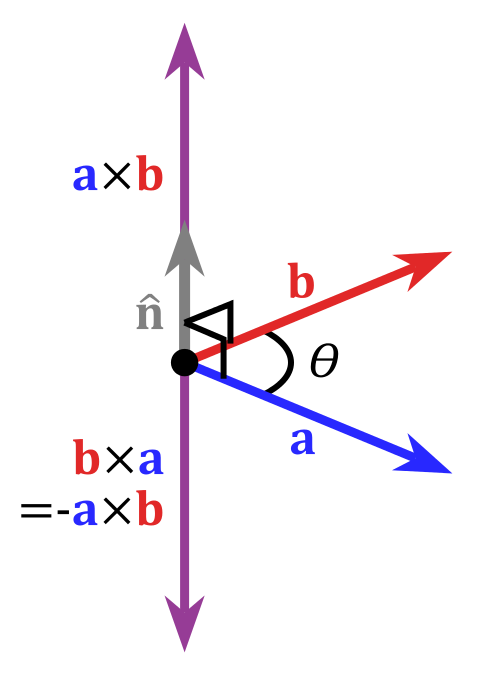特别的，在二维中，两个向量的向量积的模的绝对值等于由这两天向量组成的平行四边形的面积。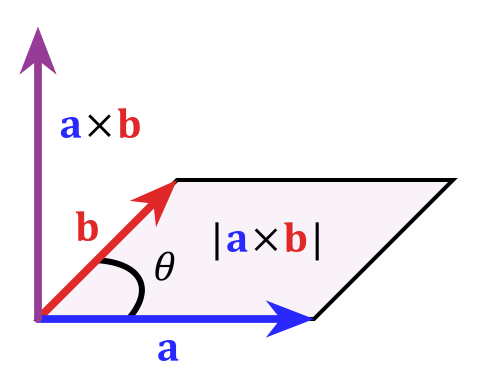参考：百度百科，维基百科

展开全文图形 向量 数学
• 对于游戏行业程序员来说，向量点乘”和“叉乘”是非常熟悉的运算。从代码上看他们很简单：（以下代码选自UE4的“Vector.h”） 点乘就是各分量逐项相乘，最终得到了一个标量： FORCEINLINE float FVector::Dot...

目标

对于游戏行业程序员来说，向量“点乘”和“叉乘”是非常熟悉的运算。从代码上看他们运算过程并不复杂：（以下代码选自UE4的“Vector.h”）

点乘就是各分量逐项相乘，最终得到了一个标量

FORCEINLINE float FVector::DotProduct(const FVector& A, const FVector& B)
{
return X*V.X + Y*V.Y + Z*V.Z;
}

叉乘最终得到一个新的向量，虽然其运算现在看起来略显“奇怪”（不过，在随后的证明中可以看出其重要的几何意义）：

FORCEINLINE FVector FVector::CrossProduct(const FVector& A, const FVector& B)
{
return FVector
(
Y * V.Z - Z * V.Y,
Z * V.X - X * V.Z,
X * V.Y - Y * V.X
);
}

即：
a ⃗ ⋅ b ⃗ = x a x b + y a y b + z a z b \vec{a}\cdot\vec{b} = x_ax_b+y_ay_b+z_az_b

a ⃗ × b ⃗ = ( y a z b − z a y b , z a x b − x a z b , x a y b − y a x b ) \vec{a}\times\vec{b} = (y_az_b-z_ay_b,z_ax_b-x_az_b,x_ay_b-y_ax_b)

他们各自都有重要的几何意义，经常会出现在有关空间计算的逻辑中，例如：

• 标准化后向量点乘得到的值为夹角的余弦。这样，只要计算点乘，得到-1~ 0 ~1，便可知道两个向量之间方向的关系是相反 ~ 垂直 ~ 相同
• 向量叉乘后得到的新向量一定和原先两个向量垂直。
• 向量叉乘后得到的向量的模，其值为两个向量构成的三角形的面积的二倍。

向量点乘和叉乘的这些特性经常被使用，但是对于其中的数学原理我是模糊不清的，因此我想自己动手证明一下。

而为了让这些证明得更容易，我还需要在此之前证明其基于的一些定理。

1. 证明：勾股定理

定理：
对于一个直角三角形ABC，其中直角为∠BAC，有：
B C 2 = A B 2 + A C 2 BC^2=AB^2+AC^2

其证明网上有很多种，下面的方法据说来自于欧几里得的《几何原本》。（图片来源勾股定理_百度百科

由于 三角形FBC正方形GFBA 同底等高，所以有：
S F B C × 2 = S G F B A S_{FBC}\times2=S_{GFBA}

由于 三角形FBC三角形ABD 为全等三角形，所以有：
S F B C = S A B D S_{FBC}=S_{ABD}

由于 三角形ABD矩形BDLK 同底等高，所以有：
S A B D × 2 = S B D L K S_{ABD}\times2=S_{BDLK}

因此：
S G F B A = S F B C × 2 = S A B D × 2 = S B D L K S_{GFBA}=S_{FBC}\times2=S_{ABD}\times2=S_{BDLK}

同理：
S A C I H = S K L E C S_{ACIH}=S_{KLEC}

（即图中粉色正方形的面积等于粉色矩形的面积，蓝色正方形的面积等于蓝色矩形的面积）

因此：
B C 2 = S B D E C = S B D L K + S K L E C = S G F B A + S A C I H = A B 2 + A C 2 \begin{aligned} BC^2 & =S_{BDEC}\\ & =S_{BDLK}+S_{KLEC}\\ & =S_{GFBA}+S_{ACIH}\\ & = AB^2+AC^2\\ \end{aligned}

2. 证明：余弦定理

定理：
对于任意一个三角形ABC，有：
A B 2 = B C 2 + A C 2 − 2 B C ⋅ A C ⋅ c o s C AB^2=BC^2+AC^2-2BC \cdot AC \cdot cosC

证明网上也有很多种，下面的方法据说也来自于欧几里得的《几何原本》。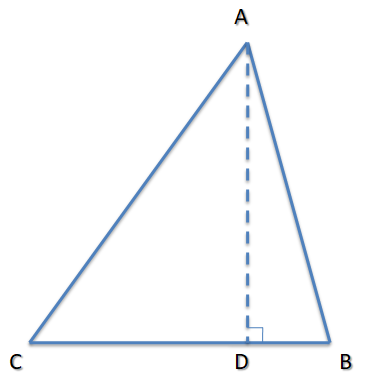A D = A C ⋅ s i n C AD = AC \cdot sinC

C D = A C ⋅ c o s C CD = AC \cdot cosC

在CB边上，有：
D B = B C − C D = B C − A C ⋅ c o s C DB= BC-CD=BC- AC \cdot cosC

A B 2 = A D 2 + D B 2 = ( A C ⋅ s i n C ) 2 + ( B C − A C ⋅ c o s C ) 2 = A C 2 ⋅ s i n C 2 + B C 2 + A C 2 ⋅ c o s C 2 − 2 B C ⋅ A C ⋅ c o s C = B C 2 + A C 2 ⋅ s i n C 2 + A C 2 ⋅ c o s C 2 − 2 B C ⋅ A C ⋅ c o s C = B C 2 + A C 2 ( s i n C 2 + c o s C 2 ) − 2 B C ⋅ A C ⋅ c o s C = B C 2 + A C 2 − 2 B C ⋅ A C ⋅ c o s C \begin{aligned} AB^2 & =AD^2+DB^2\\ & =(AC \cdot sinC)^2+(BC- AC \cdot cosC)^2\\ & =AC^2\cdot sinC^2+BC^2+AC^2\cdot cosC^2-2BC \cdot AC \cdot cosC\\ & =BC^2+AC^2\cdot sinC^2+AC^2\cdot cosC^2-2BC \cdot AC \cdot cosC\\ & =BC^2+AC^2(sinC^2+cosC^2)-2BC \cdot AC \cdot cosC\\ & =BC^2+AC^2-2BC \cdot AC \cdot cosC\\ \end{aligned}

3. 证明：向量点乘的几何意义——结果为模相乘再乘夹角余弦

向量点乘的定义如下：
a ⃗ ⋅ b ⃗ = x a x b + y a y b + z a z b \vec{a}\cdot\vec{b} = x_ax_b+y_ay_b+z_az_b

现在想证明的是：（其中θ为两向量夹角）
a ⃗ ⋅ b ⃗ = ∥ a ⃗ ∥ ⋅ ∥ b ⃗ ∥ c o s θ \vec{a}\cdot\vec{b} =\left \| \vec{a} \right \|\cdot\left \| \vec{b} \right \|cos\theta

在证明前，先看一个算不上是“定理”的结论：
对于一个2维的向量(x,y)，由于坐标系是垂直的，所以由勾股定理很容易能推导出
2 维 向 量 长 度 2 = x 2 + y 2 2维向量长度^2=x^2+y^2

对于一个3维的向量(x,y,z)，也可以很容易能推导出：
3 维 向 量 长 度 2 = x 2 + y 2 + z 2 3维向量长度^2=x^2+y^2+z^2

下面正式开始证明：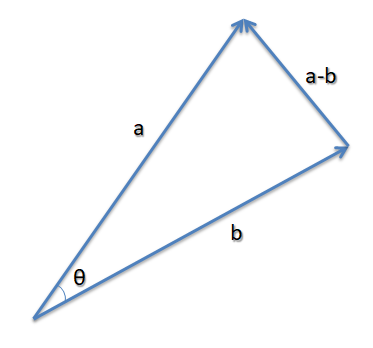如果将向量a和向量b的起点放在一起，那么这两个向量终点之间的向量即为 a-b

而根据余弦定理，有：
∥ a ⃗ − b ⃗ ∥ 2 = ∥ a ⃗ ∥ 2 + ∥ b ⃗ ∥ 2 − 2 ∥ a ⃗ ∥ ⋅ ∥ b ⃗ ∥ c o s θ \left \| \vec{a} -\vec{b} \right \|^2=\left \| \vec{a} \right \|^2 +\left \| \vec{b} \right \|^2 -2\left \| \vec{a} \right \|\cdot\left \| \vec{b} \right \|cos\theta
写成分量的形式，就是：
( ( x a , y a , z a ) − ( x b , y b , z b ) ) 2 = ( x a , y a , z a ) 2 + ( x b , y b , z b ) 2 − 2 ∥ a ⃗ ∥ ⋅ ∥ b ⃗ ∥ c o s θ ((x_a,y_a,z_a)-(x_b,y_b,z_b))^2=(x_a,y_a,z_a)^2+(x_b,y_b,z_b)^2-2\left \| \vec{a} \right \|\cdot\left \| \vec{b} \right \|cos\theta

向量相减即各分量相减，即：
( x a − x b , y a − y b , z a − z b ) 2 = ( x a , y a , z a ) 2 + ( x b , y b , z b ) 2 − 2 ∥ a ⃗ ∥ ⋅ ∥ b ⃗ ∥ c o s θ (x_a-x_b,y_a-y_b,z_a-z_b)^2=(x_a,y_a,z_a)^2+(x_b,y_b,z_b)^2-2\left \| \vec{a} \right \|\cdot\left \| \vec{b} \right \|cos\theta

将平方的运算展开：
x a 2 + x b 2 − 2 x a x b + y a 2 + y b 2 − 2 y a y b + z a 2 + z b 2 − 2 z a z b = x a 2 + x b 2 + y a 2 + y b 2 + z a 2 + z b 2 − 2 ∥ a ⃗ ∥ ⋅ ∥ b ⃗ ∥ c o s θ x_a^2+x_b^2-2x_ax_b+y_a^2+y_b^2-2y_ay_b+z_a^2+z_b^2-2z_az_b=x_a^2+x_b^2+y_a^2+y_b^2+z_a^2+z_b^2-2\left \| \vec{a} \right \|\cdot\left \| \vec{b} \right \|cos\theta

去掉等式两边重复项可得：
− 2 x a x b − 2 y a y b − 2 z a z b = − 2 ∥ a ⃗ ∥ ⋅ ∥ b ⃗ ∥ c o s θ -2x_ax_b-2y_ay_b-2z_az_b=-2\left \| \vec{a} \right \|\cdot\left \| \vec{b} \right \|cos\theta

约去-2得：
x a x b + y a y b + z a z b = ∥ a ⃗ ∥ ⋅ ∥ b ⃗ ∥ c o s θ x_ax_b+y_ay_b+z_az_b=\left \| \vec{a} \right \|\cdot\left \| \vec{b} \right \|cos\theta

结合向量点乘的定义，则最后可知：
a ⃗ ⋅ b ⃗ = x a x b + y a y b + z a z b = ∥ a ⃗ ∥ ⋅ ∥ b ⃗ ∥ c o s θ \begin{aligned} \vec{a}\cdot\vec{b} & =x_ax_b+y_ay_b+z_az_b \\ & =\left \| \vec{a} \right \|\cdot\left \| \vec{b} \right \|cos\theta \\ \end{aligned}

4. 证明：向量叉乘的几何意义——结果与原先两个向量都垂直

向量叉乘的定义如下：
a ⃗ × b ⃗ = ( y a z b − z a y b , z a x b − x a z b , x a y b − y a x b ) \vec{a}\times\vec{b} = (y_az_b-z_ay_b,z_ax_b-x_az_b,x_ay_b-y_ax_b)

由前面证明的向量点乘的几何意义可知，
如果能证明：

( a ⃗ × b ⃗ ) ⋅ a ⃗ = 0 (\vec{a}\times\vec{b} )\cdot \vec{a}=0

( a ⃗ × b ⃗ ) ⋅ b ⃗ = 0 (\vec{a}\times\vec{b} )\cdot \vec{b}=0

则意味着向量叉乘的结果向量，和原先两个向量的夹角的余弦值都为0，即夹角为90°，即与原先两个向量都垂直

而这个证明可以直接从算式中得出：
( a ⃗ × b ⃗ ) ⋅ a ⃗ = ( y a z b − z a y b , z a x b − x a z b , x a y b − y a x b ) ⋅ ( x a , y a , z a ) = y a z b x a − z a y b x a + z a x b y a − x a z b y a + x a y b z a − y a x b z a = ( y a z b x a − x a z b y a ) + ( x a y b z a − z a y b x a ) + ( z a x b y a − y a x b z a ) = 0 + 0 + 0 = 0 \begin{aligned} (\vec{a}\times\vec{b} )\cdot \vec{a} & = (y_az_b-z_ay_b,z_ax_b-x_az_b,x_ay_b-y_ax_b) \cdot (x_a,y_a,z_a) \\ & =y_az_bx_a-z_ay_bx_a+z_ax_by_a-x_az_by_a+x_ay_bz_a-y_ax_bz_a\\ & =(y_az_bx_a-x_az_by_a)+(x_ay_bz_a-z_ay_bx_a)+(z_ax_by_a-y_ax_bz_a)\\ & =0+0+0\\ & =0\\ \end{aligned}

( a ⃗ × b ⃗ ) ⋅ b ⃗ = ( y a z b − z a y b , z a x b − x a z b , x a y b − y a x b ) ⋅ ( x b , y b , z b ) = y a z b x b − z a y b x b + z a x b y b − x a z b y b + x a y b z b − y a x b z b = ( y a z b x b − y a x b z b ) + ( z a x b y b − z a y b x b ) + ( x a y b z b − x a z b y b ) = 0 + 0 + 0 = 0 \begin{aligned} (\vec{a}\times\vec{b} )\cdot \vec{b} & = (y_az_b-z_ay_b,z_ax_b-x_az_b,x_ay_b-y_ax_b) \cdot (x_b,y_b,z_b) \\ & =y_az_bx_b-z_ay_bx_b+z_ax_by_b-x_az_by_b+x_ay_bz_b-y_ax_bz_b\\ & =(y_az_bx_b-y_ax_bz_b)+(z_ax_by_b-z_ay_bx_b)+(x_ay_bz_b-x_az_by_b)\\ & =0+0+0\\ & =0\\ \end{aligned}

5. 证明：向量叉乘的几何意义——结果的模为原先两向量的模相乘再乘夹角正弦

向量叉乘的定义如下：
a ⃗ × b ⃗ = ( y a z b − z a y b , z a x b − x a z b , x a y b − y a x b ) \vec{a}\times\vec{b} = (y_az_b-z_ay_b,z_ax_b-x_az_b,x_ay_b-y_ax_b)

现在想证明的是：（其中θ为两向量夹角）
∥ a ⃗ × b ⃗ ∥ = ∥ a ⃗ ∥ ∥ b ⃗ ∥ s i n θ \left \|\vec{a}\times\vec{b}\right \| =\left \| \vec{a} \right \|\left \| \vec{b} \right \|sin\theta

（方法来自于《3D游戏与计算机图形学中的数学方法》）

取向量叉乘结果的平方，逐步展开：
∥ a ⃗ × b ⃗ ∥ 2 = ( y a z b − z a y b , z a x b − x a z b , x a y b − y a x b ) 2 = ( y a z b − z a y b ) 2 + ( z a x b − x a z b ) 2 + ( x a y b − y a x b ) 2 = ( y a 2 z b 2 + z a 2 y b 2 − 2 y a z b z a y b ) + ( z a 2 x b 2 + x a 2 z b 2 − 2 z a x b x a z b ) + ( x a 2 y b 2 + y a 2 x b 2 − 2 x a y b y a x b ) = ( y a 2 z b 2 + z a 2 y b 2 + z a 2 x b 2 + x a 2 z b 2 + x a 2 y b 2 + y a 2 x b 2 ) + ( − 2 y a z b z a y b − 2 z a x b x a z b − 2 x a y b y a x b ) \begin{aligned} \left \|\vec{a}\times\vec{b}\right \| ^2 & = (y_az_b-z_ay_b,z_ax_b-x_az_b,x_ay_b-y_ax_b)^2 \\ & = (y_az_b-z_ay_b)^2+(z_ax_b-x_az_b)^2+(x_ay_b-y_ax_b)^2 \\ & = (y_a^2z_b^2+z_a^2y_b^2-2y_az_bz_ay_b)+(z_a^2x_b^2+x_a^2z_b^2-2z_ax_bx_az_b)+(x_a^2y_b^2+y_a^2x_b^2-2x_ay_by_ax_b) \\ & = (y_a^2z_b^2+z_a^2y_b^2+z_a^2x_b^2+x_a^2z_b^2+x_a^2y_b^2+y_a^2x_b^2)+(-2y_az_bz_ay_b-2z_ax_bx_az_b-2x_ay_by_ax_b)\\ \end{aligned}

下面便出现了我觉得这种证明方法比较“魔法”的一个操作，对于等号右侧：
左部分先加上了 ( x a 2 x b 2 + y a 2 y b 2 + z a 2 z b 2 ) (x_a^2x_b^2+y_a^2y_b^2+z_a^2z_b^2) ，右部分再减去它。一番“折腾”之后，虽然结果未受影响，但是却让左部分凑出了原先两向量的模的形式，右部分凑出了向量点乘的形式，具体来看：
∥ a ⃗ × b ⃗ ∥ 2 = ( y a 2 z b 2 + z a 2 y b 2 + z a 2 x b 2 + x a 2 z b 2 + x a 2 y b 2 + y a 2 x b 2 ) + ( x a 2 x b 2 + y a 2 y b 2 + z a 2 z b 2 ) + ( − 2 y a z b z a y b − 2 z a x b x a z b − 2 x a y b y a x b ) − ( x a 2 x b 2 + y a 2 y b 2 + z a 2 z b 2 ) = ( y a 2 z b 2 + z a 2 y b 2 + z a 2 x b 2 + x a 2 z b 2 + x a 2 y b 2 + y a 2 x b 2 + x a 2 x b 2 + y a 2 y b 2 + z a 2 z b 2 ) − ( x a 2 x b 2 + y a 2 y b 2 + z a 2 z b 2 + 2 y a z b z a y b + 2 z a x b x a z b + 2 x a y b y a x b ) = ( x a 2 + y a 2 + z a 2 ) ( x b 2 + y b 2 + z b 2 ) − ( x a x b + y a y b + z a z b ) 2 = ∥ a ⃗ ∥ 2 ∥ b ⃗ ∥ 2 − ( a ⃗ ⋅ b ⃗ ) 2 \begin{aligned} \left \|\vec{a}\times\vec{b}\right \| ^2 & = (y_a^2z_b^2+z_a^2y_b^2+z_a^2x_b^2+x_a^2z_b^2+x_a^2y_b^2+y_a^2x_b^2)+(x_a^2x_b^2+y_a^2y_b^2+z_a^2z_b^2)+(-2y_az_bz_ay_b-2z_ax_bx_az_b-2x_ay_by_ax_b)-(x_a^2x_b^2+y_a^2y_b^2+z_a^2z_b^2)\\ & = (y_a^2z_b^2+z_a^2y_b^2+z_a^2x_b^2+x_a^2z_b^2+x_a^2y_b^2+y_a^2x_b^2+x_a^2x_b^2+y_a^2y_b^2+z_a^2z_b^2)-(x_a^2x_b^2+y_a^2y_b^2+z_a^2z_b^2+2y_az_bz_ay_b+2z_ax_bx_az_b+2x_ay_by_ax_b)\\ & = (x_a^2+y_a^2+z_a^2)(x_b^2+y_b^2+z_b^2)-(x_ax_b+y_ay_b+z_az_b)^2\\ & =\left \| \vec{a} \right \|^2\left \| \vec{b} \right \|^2-(\vec{a}\cdot\vec{b})^2\\ \end{aligned}

而根据前面证明的向量点乘的几何意义可知：
a ⃗ ⋅ b ⃗ = ∥ a ⃗ ∥ ⋅ ∥ b ⃗ ∥ c o s θ \vec{a}\cdot\vec{b} =\left \| \vec{a} \right \|\cdot\left \| \vec{b} \right \|cos\theta

所以：
∥ a ⃗ × b ⃗ ∥ 2 = ∥ a ⃗ ∥ 2 ∥ b ⃗ ∥ 2 − ∥ a ⃗ ∥ 2 ∥ b ⃗ ∥ 2 ⋅ ( c o s θ ) 2 = ∥ a ⃗ ∥ 2 ∥ b ⃗ ∥ 2 ⋅ ( 1 − ( c o s θ ) 2 ) \begin{aligned} \left \|\vec{a}\times\vec{b}\right \| ^2 & = \left \| \vec{a} \right \|^2\left \| \vec{b} \right \|^2-\left \| \vec{a} \right \|^2\left \| \vec{b} \right \|^2\cdot (cos\theta)^2\\ & = \left \| \vec{a} \right \|^2\left \| \vec{b} \right \|^2\cdot (1- (cos\theta)^2) \end{aligned}

又因为【 ( c o s θ ) 2 + ( s i n θ ) 2 = 1 (cos\theta)^2+(sin\theta)^2=1 】一定成立
所以：
∥ a ⃗ × b ⃗ ∥ 2 = ∥ a ⃗ ∥ 2 ∥ b ⃗ ∥ 2 ⋅ ( s i n θ ) 2 \left \|\vec{a}\times\vec{b}\right \| ^2= \left \| \vec{a} \right \|^2\left \| \vec{b} \right \|^2\cdot (sin\theta)^2

开方得：
∥ a ⃗ × b ⃗ ∥ = ∥ a ⃗ ∥ ∥ b ⃗ ∥ ⋅ s i n θ \left \|\vec{a}\times\vec{b}\right \|= \left \| \vec{a} \right \|\left \| \vec{b} \right \|\cdot sin\theta

6. 证明：向量叉乘的几何意义——结果的模为原先两向量构成三角形的面积二倍

在前者证明之后，此证明变得很容易，因为：

∥ b ⃗ ∥ ⋅ s i n θ \left \| \vec{b} \right \|\cdot sin\theta 的长度就是以a为底边的三角形的高度：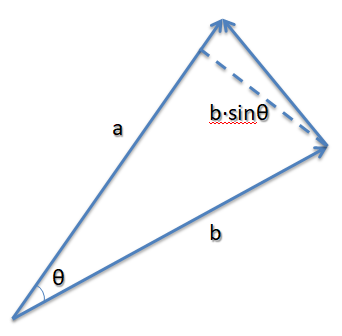所以：
∥ a ⃗ × b ⃗ ∥ = ∥ a ⃗ ∥ ∥ b ⃗ ∥ ⋅ s i n θ = ( ∥ a ⃗ ∥ ) × ( ∥ b ⃗ ∥ ⋅ s i n θ ) = 底 × 高 = 2 × ( 底 × 高 2 ) = 2 × S 三 角 形 \begin{aligned} \left \|\vec{a}\times\vec{b}\right \| & = \left \| \vec{a} \right \|\left \| \vec{b} \right \|\cdot sin\theta\\ & = (\left \| \vec{a} \right \|)\times (\left \| \vec{b} \right \|\cdot sin\theta)\\ & = 底 \times 高\\ & = 2\times (\frac{底 \times 高}{2})\\ & = 2\times S_{三角形} \end{aligned}

总结

向量点乘

定义

a ⃗ ⋅ b ⃗ = x a x b + y a y b + z a z b \vec{a}\cdot\vec{b} = x_ax_b+y_ay_b+z_az_b

几何意义与作用举例

a ⃗ ⋅ b ⃗ = ∥ a ⃗ ∥ ⋅ ∥ b ⃗ ∥ c o s θ \vec{a}\cdot\vec{b} =\left \| \vec{a} \right \|\cdot\left \| \vec{b} \right \|cos\theta

• 可以算出向量的夹角
• 可以直接根据此值判断两向量方向的关系：-1~ 0 ~1对应于相反 ~ 垂直 ~ 相同

向量叉乘

定义

a ⃗ × b ⃗ = ( y a z b − z a y b , z a x b − x a z b , x a y b − y a x b ) \vec{a}\times\vec{b} = (y_az_b-z_ay_b,z_ax_b-x_az_b,x_ay_b-y_ax_b)

几何意义与作用举例1

结果的向量与原先两个向量都垂直

• 可以用来快速算出两个向量确定的一个平面的法向量方向。
几何意义与作用举例2

∥ a ⃗ × b ⃗ ∥ = ∥ a ⃗ ∥ ∥ b ⃗ ∥ s i n θ \left \|\vec{a}\times\vec{b}\right \| =\left \| \vec{a} \right \|\left \| \vec{b} \right \|sin\theta

• 可以用来在已知顶点位置情况下，快速算出空间内一个三角形的面积。
展开全文数学
• 向量点乘（内积） 向量点乘公式为： ...内积（点乘）的几何意义： 表征或计算两个向量之间的夹角 b向量在a向量方向上的投影 判断两个向量是否同一方向或正交(即垂直)等方向关系，具体对应关系为：...

向量点乘（内积）

向量点乘公式为：

a * b = |a| * |b| * cosθ

点乘的结果是是标量点乘也被称为内积，是a向量在b向量上投影的长度与b向量的长度的乘积，反映了两个向量之间的相似度，两向量越相似，它们的点积就越大。

内积（点乘）的几何意义：

1. 表征或计算两个向量之间的夹角
2. b向量在a向量方向上的投影

判断两个向量是否同一方向或正交(即垂直)等方向关系，具体对应关系为：

a∙b>0→方向基本相同，夹角在0°到90°之间
a∙b=0→ 正交，相互垂直
a∙b<0→ 方向基本相反，夹角在90°到180°之间

向量叉乘（外积）

向量叉乘公式为：

a ^ b = |a| * |b| * sinθ

叉乘的结果是一个新的向量，所以也称为向量积，它垂直于相乘的a、b两向量所构成的平面。

外积（叉乘）的几何意义：

在三维几何中，向量a和向量b的外积结果是一个向量，有个更通俗易懂的说法是法向量，该向量垂直于a和b向量构成的平面。

在3D图像学中，外积的概念非常有用，可以通过两个向量的外积，生成第三个垂直于a，b的法向量，从而构建X、Y、Z坐标系。如下图所示：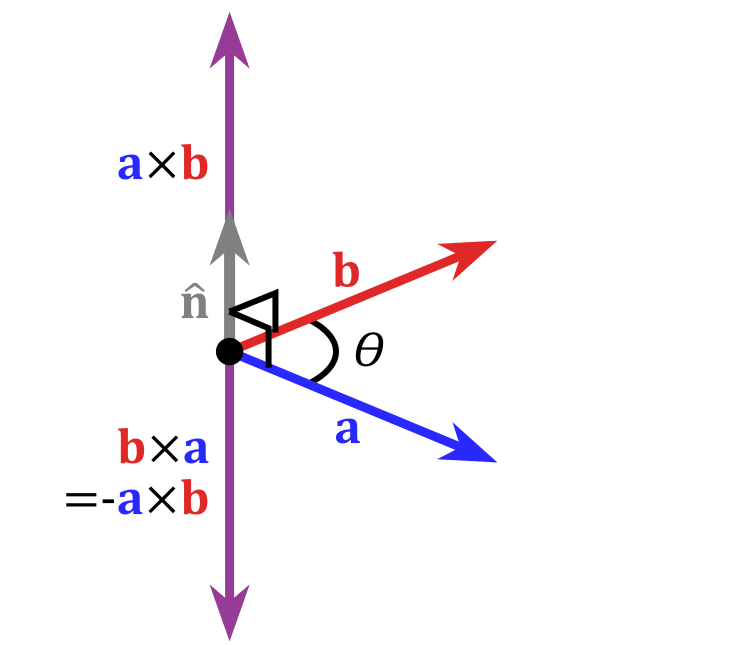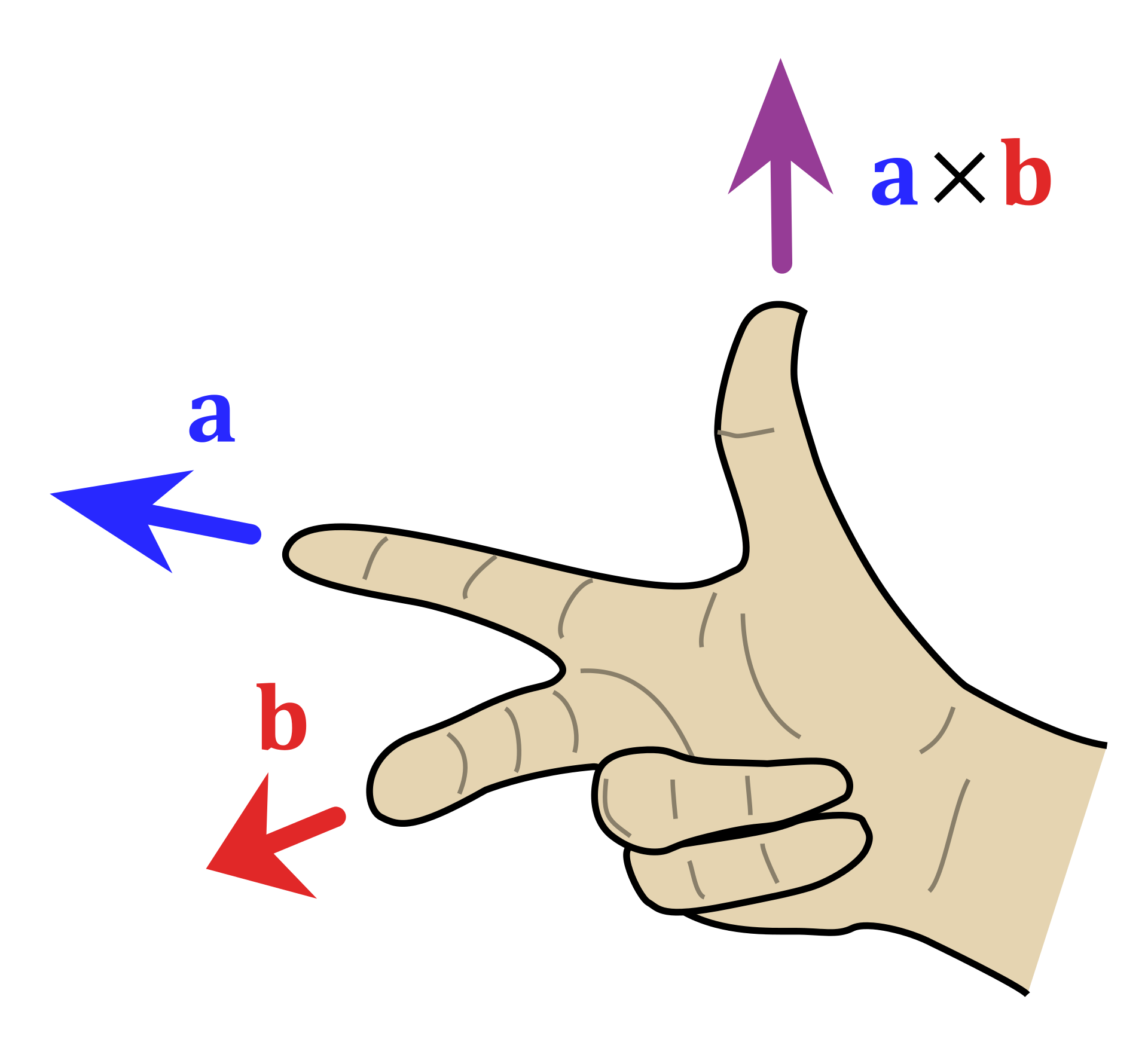可以通过右手定则确定叉乘的方向

在游戏开发中，可以使用叉乘，确定敌方目标在玩家左右方向。

假设玩家的正方向是a向量，b是玩家指向敌方目标的向量，叉乘方向向上代表目标在玩家左边。反之，叉乘方向向下代表目标在玩家右边。

展开全文向量点积 向量叉乘
• 向量是由n个实数组成的一个n行1列（n*1）或一个1行n列（1*n）的有序数组； 向量点乘,也叫向量的内积、数量积，对两个向量执行点乘运算，就是对这两个向量对应位一一相乘之后求和的操作，点乘...对于向量a向量b向量点乘 向量积 法向量 内积
• 向量是由n个实数组成的一个n行1列（n*1）或一个1行n列（1*n）的有序数组；...向量点乘,也叫向量的内积、数量积，对两个向量执行点乘运算，就是对这两个向量对应位一一相乘之后求和的操作，点...向量 点乘 内积
• 向量的点乘和叉乘

千次阅读 2021-03-07 10:36:29
向量点乘和叉乘2019-12-09 15:46:24文/董月点乘，也叫...点乘点乘的结果是一个实数a·b=|a|·|b|·cos叉乘：叉乘的结果是一个向量几何意义点乘几何意义可以用来表征或计算两个向量之间的夹角，以及在b向量在...
• 向量的点乘（一）

万次阅读 2019-05-18 10:46:52
向量点乘（一） 1.点乘的定义 向量u和向量v之间最小的夹角我们记做为[u,v],如下图所示，两个向量之间的夹角我们用绿色弧形表示，其中一个夹角用绿色矩形表示，这意味着这两个向量的夹角为90度或者是π/2，即[u,v...线性代数 点乘 向量
• 声明： 本文转自这里 向量是由n个实数组成的一个n行1列（n*1）或一个1行n列（1*n）的有序数组； 向量点乘,也叫向量的内积、数量积，对...对于向量a向量b： &nbsp; &nbsp; &nbsp; &...向量 点乘
•线性代数
• 1.向量点乘公式推导和几何解释 01.向量点乘(dot product)是其各个分量乘积的和，公式： 用连加号写： 02.几何解释： 点乘的结果是一个标量，等于向量大小与夹角的cos值的乘积。 ab = |a||b|cosθ 如果a...
• 向量点乘（内积）和叉乘（外积、向量积）概念及几何意义解读 向量是由n个实数组成的一个n行1列（n*1）或一个1行n列（1*n）的有序数组； 向量的点乘,也叫向量的内积、数量积，对两个向量执行点乘运算，就是对这两...
• 【转】向量点乘(内积)和叉乘(外积、向量积)概念及几何意义解读向量是由n个实数组成的一个n行1列(n*1)或一个1行n列(1*n)的有序数组；向量的点乘,也叫向量的内积、数量积，对两个向量执行点乘运算，就是对这两个向量...
• Unity中点乘和叉乘点乘（API: Vector3.Dot()）点乘的计算公式点乘几何意义用途之一：判断一个物体当前方位利用点乘求出角度叉乘（API: Vector3.Cross()）叉乘计算公式叉乘的几何意义判断物体是在左侧还是右侧 点乘...
• 二、应用不同：1、点乘：平面向量的数量积a·b是一个非常重要的概念，利用它可以很容易地证明平面几何的许多命题，例如勾股定理、菱形的对角线相互垂直、矩形的对角线相等等。2、在物理学光学和计算机图形学中，叉积...
• 向量点乘:a * b 公式：a * b = |a| * |b| * cosθ  点乘又叫向量的内积、数量积，是一个向量和它在另一个向量上的投影的长度的乘积；是标量。  点乘反映着两个向量的“相似度”，两个向量越“相似”，它们...
• 参考： https://blog.csdn.net/dcrmg/article/details/52416832 ... 1 向量内积（点乘） ...ab的点积（点乘）公式为： ...向量内积的几何意义及用途 包括： 表征或计算两个向量之间的夹角 b向量a向量...向量
• 对两个向量执行点乘运算，就是对这两个向量对应位一一相乘之后求和的操作，如下所示，对于向量a向量bab的点积公式为： 这里要求一维向量a向量b的行列数相同。注意：点乘的结果是一个标量(数量而不是...内积
• 向量点乘a * b（常被写为a· b） 点乘,也叫向量的内积、数量积.顾名思义,求下来的结果是一个数. 向量a·向量b=|a||b|cos 在物理学中,已知力与位移求功,实际上就是求向量F与向量s的内积,即要用点乘. 向量叉乘：a ...线性代数
• 向量点乘与向量叉乘的意义

万次阅读 2018-08-15 15:47:27
这是博客原文：向量点乘与叉乘的几何意义。我主要是为了方便自已以后添加和查找。 向量的点积公式为：a * b = |a| * |b| * cosθ，点积的结果是数量而不是向量所以点积也被称为数量积或者内积，是a向量在b向量上......